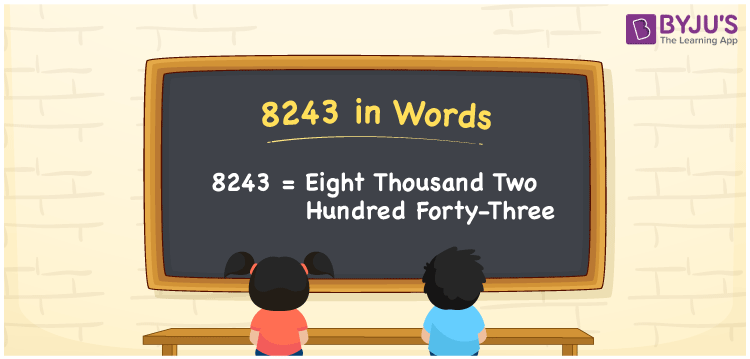# 8243 in Words

The number 8243 is written as “Eight thousand two hundred forty-three”. The number 8243 is a 4 digit number and it lies after the number 8242 but before 8244. It is also a cardinal number. For example, if you purchased the books for Rs. 8243, it can be written as “I purchased the books for Rs. Eight thousand two hundred forty-three”.

 8243 in Words: Eight Thousand Two Hundred Forty-three. Eight Thousand Two Hundred Forty-three in Numerical Form: 8243.

## 8243 in English Words## How to Write 8243 in Words?

The following table represents the place values of the number 8243.

 Thousands Hundreds Tens Ones 8 2 4 3

The expanded form of 8243 is as follows:

= 8 × Thousand + 2 × Hundred + 4 × Ten + 3 × One

= 8 × 1000 + 2 × 100 + 4 × 10 + 3 × 1

= 8000 + 200 + 40 + 3

= 8243

= Eight thousand two hundred forty-three

Hence, 8243 in words is eight thousand two hundred forty-three.

8243 in words – Eight thousand two hundred forty-three

Is 8243 an odd number? – Yes

Is 8243 an even number? – No

Is 8243 a perfect square number? – No

Is 8243 a perfect cube number? – No

Is 8243 a prime number? – Yes

Is 8243 a composite number? – No

## Frequently Asked Questions on 8243 in Words

Q1

### How to write 8243 in words?

8243 in words is eight thousand two hundred forty-three.

Q2

### Simplify 8000 + 243, and express it in words.

Simplifying 8000 + 243, we get 8243. Hence, 8243 in words is eight thousand two hundred forty-three.

Q3

### Is 8243 a prime number?

Yes, 8243 is a prime number.